Next stop, radical town. All aboard the root train. While en route, all food service will be suspended with the exception of simplifying square roots and imaginary numbers.Okay, Shmoopers. We admit it. That was corny. And we know that you can't eat square roots or imaginary numbers. We were just getting tired of the radical surfer references. Regardless, this section is all about roots and radicals.

A radical is any expression that uses the √ symbol. Take ∛5, for instance. This is a radical expression. In this expression, 3 is technically called the index, √ is known as the radical symbol, and 5 is the radicand.

The next thing we need to know is how to simplify one of these so-called radicals. In other words, how to write a radical in what's otherwise known as simple radical form (more on that later). From there, our lovely sample problems will help us learn how to add, subtract, multiply, and divide roots. Enjoy the Shmooptastic ride.

### Sample Problem

Simplify the following: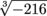.

This is just a bit of a warm-up. We're reminding you that more than just square roots do exist. This cube root is really asking the question: What number multiplied by itself 3 times is -216?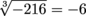Nailed it. The answer's -6, because (-6)(-6)(-6) = -216.

### Sample Problem

Simplify the following: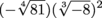.

This one's still pretty basic, despite there being a couple steps to navigate. First, let's evaluate these radicals in the parentheses.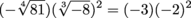Next, we should follow our order of operations and square the negative 2.

(-3)(-2)2 = (-3)(4)

Lastly, let's multiply and move on with our lives.

(-3)(4) = -12

### Sample Problem

Simplify the following: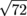.

This problem isn't quite so easy;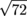doesn't simplify to some nice, neat whole number like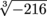did in the first sample problem. That means we need to break down the radical into simple radical form. To do this, we break downinto a product of prime numbers.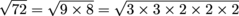Once we have our prime number breakdown in the bag, we can start to simplify. Since √(3 × 3) = 3, we can pull a 3 out of the square root. Gee, we can pull out a 2 as well. This gives us…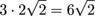This is simple radical form. In other words, our radical now contains only a product of unique primes. The key idea to remember is that when you have a pair of primes, you can always pull one out.

### Sample Problem

Simplify the following: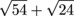.

This is a little bit tougher. Don't worry. We have you covered. All we really need to do is break down both square roots into their simple radical form.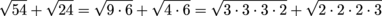Next, we're going to pull out our pairs.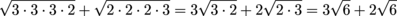By the way, if you get comfortable and go right from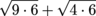to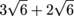, go for it. That just means that you're starting to truly understand, grasshopper.

Finally, since we've got two like terms, we can add them together. This might be confusing, but it's really no different than saying 3x + 2x = 5x. The difference here is that instead of x, we're using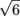.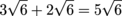One final note: adding like terms such as this is only okay if the terms are really alike. You wouldn't add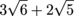together, for the same reason you wouldn't add 3x + 2y together. Understand, rubber band?

So, you think you've conquered exponent rules, mastered negative exponents, and even shown radicals who's boss? Then why not give fractional exponents a try?

Question: When do we use fractional exponents?

Answer: We can write any radical expression using a fractional exponent. A simple equation like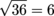is literally the same as 361/2 = 6. Seriously. They mean exactly the same thing. This also means that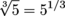and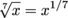.

While this may be a breakthrough of epic proportions, there is much more. Not only can we write radicals using fractional exponents, we can also combine indices with an exponent.

FYI: "Indices" is plural for index.

So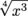is the same as x3/4, and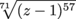is the same as (z – 1)57/71. Just stick the index of the radical in the denominator, with the original exponent in the numerator. This is useful because now we can apply all our exponent rules to radicals, too. Scizzore.

### Sample Problem

Simplify the following: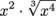.

First thing we're going to do is rewrite this equation using only exponents. Fractions, here we come.

x2x4/3

Next, we know we've got to add exponents. This means we'll need to make sure 2 is rewritten as 6/3 before we add things up for our final answer.

x2x4/3 = x6/3x4/3 = x10/3

Annnnd, we're done. For now.

### Sample Problem

Simplify the following: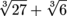.

Moving left to right, let's get our Shmoop on. The first term is easy.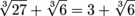The next term can't readily be simplified, unless we'd like to write it using a fractional exponent.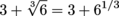The question now is: Are we able to add 3 and 61/3? Whenever we run into a problem like this, our advice is to always compare it to another, more familiar situation. For instance, can x + y2 be simplified? Of course not. Problem solved.

### Sample Problem

Simplify the following: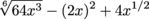.

It's back to the grind. This one forces us to move term by term once again. The whole number in the first term isn't too tough. However, the exponent is going to require some fractionalization. Yes, we did just make up a word.

2(x3)1/6 – (2x)2 + 4x1/2 = 2x3/6 – (2x)2 + 4x1/2

Simplifying the 36 exponent to ½ while simplifying that middle term gives us:

2x3/6 – (2x)2 + 4x1/2 = 2x1/2 – 4x2 + 4x1/2

What's nice here is that we can now add the like terms. Be careful; in this case, like terms are only those whose x factors have the same exponents.

2x1/2 – 4x2 + 4x1/2 = 6x1/2 – 4x2

That's as simple as we can make it.

### Sample Problem

Simplify the following using positive exponents: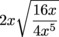.

Let's start at the beginning. For this problem, that means we'll start by simplifying things inside of that square root.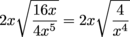Next, we can take the square root of the numerator and denominator separately. Notice how the denominator will use our fractional exponent rules.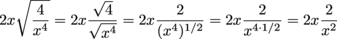Next, we can multiply. Remember, it's really like the 2x is over an invisible 1. This makes our multiplication no different than regular old fraction multiplication.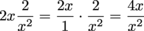Last but not least, we need to take care of the x's we have in the numerator and denominator. This is just a classic case of negative exponents. No sweat.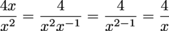Of course, we're going to great lengths to show you every single step. If your Shmoopability is on the fast track, feel free to skip a step here or there. Eventually, it'll be no big deal if your actual work for this problem looks like: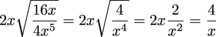### Rationalizing the Denominator. (Yes, We're Serious.)

Rationalizing the denominator always sounds like something that might be done at NASA just before the space station takes off. That's not the case. Allow us to break things down to size.Rationalizing is simply the process of making sure a number is actually a rational number. A rational number is just a number that can be written as a fraction. For instance 2, ¾, 17.12, and 1,000,000 are all rational numbers. On the other hand, π and √2 are both irrational. No matter how hard we try, we can't write them as fractions.

Therefore, it would make sense that rationalizing the denominator is really just the process of writing the denominator as a rational number. The question remains: How?

### Sample Problem

Simplify the following completely: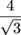.

To get this guy into its simplest form, we need to rationalize the denominator. Mathematicians hate, just hate, when there's a loose square root on the bottom of a fraction. Let's do them a solid and get rid of it.

Step one is to multiply our fraction by some form of 1. That form is determined by the square root in the denominator. Here it will be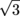. After all,overis just equal to 1. See what we're talking about?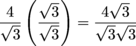Since×is 3, we can simplify and finish up.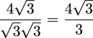Don't fret. It's no sweat. Are we done yet?

### Sample Problem

Simplify the following completely: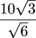.

Sometimes there's a bit of simplification that occurs after the denominator has been rationalized. That will happen here. But first…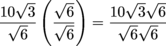After we multiply by our giant 1, we can multiply within our square roots.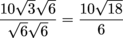At this point, we actually have two more simplifications. We know that the fraction 106 reduces to 53. But first, we can actually simplify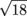.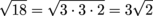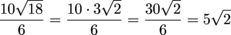### Sample Problem

Simplify the following completely: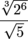.

While this problem may look complicated, our fractional exponent skills can go a long way here. Let's start by simplifying the numerator.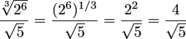Next, we need to rationalize the denominator just as we've done before.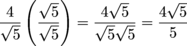While it may not look extremely simplified, this is the best this thing is going to get.

### Sample Problem

Simplify the following: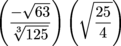.

This problem has a lot going on. First, we notice that we can actually solve a few of these radicals straight away. In face, we'll solve every one except for the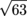.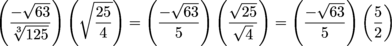This helps a great deal. Next, we can deal with theby writing it in simple radical form. For now, we'll leave everything else alone.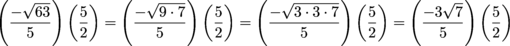Finally, we can multiply fractions across the top and bottom while dividing out 5 over 5…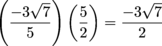If you'd like, you can also write your final answer as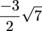. Either way, it's time to move on.